# Intraclass Correlation:

For Unordered Pairs

David C. HowellI was recently asked a question to which I gave an inadequate answer, so this page is an attempt at correcting that failing. The problem concerns calculating a correlation between two variables when it is not clear which variable should be X or Y for a given row of data. The simplest, and most common, solution is to use an intraclass correlation coefficient.

There are a number of different intraclass correlations, and the classic reference is Shrout and Fleiss (1979). The reference to Griffin and Gonzales (1995), given below, is another excellent source. I tend to think of intraclass correlations as either measures of reliability or measures of the magnitude of an effect, but they have an equally important role when it comes to calculating the correlations between pairs of observations that don't have an obvious order.

If we are using a standard Pearson correlation, we have two columns of data whose membership is clear. For example, one column might be labeled height and the other weight, and it is obvious that the person's height goes in the first column and weight in the second. If you wanted to ask if there was a correlation between the weights of husbands and wives, you would have a column labeled Husband, and one labeled Wife, and again it is obvious which is which.  But suppose that you are studying the weights of partners in gay couples. Which partner would go in which column? You could label the columns Partner1 and Partner2, but there is no obvious way to decide which partner is which. The same thing commonly happens when people are doing twin studies.

The answer here is that you cannot calculate a meaningful Pearson correlation, because you would have a different correlation if you are reversed the Partner1-Partner2 assignment of one or more of the pairs, and assignments are arbitrary. So what do you do?

When I was first asked this question I was in the midst of playing with resampling techniques. To someone with a hammer, everything looks like a nail, and to someone with a resampling program, everything looks like a resampling problem. So what I did was to write a small program that randomly assigned members of each gay couple to Partner1 or Partner2, calculated the correlation, redid the random assignment and recalculated the correlation, etc. This left me with the sampling distribution of the correlation coefficient under random resampling, and I could calculate a mean correlation and confidence limits on that correlation. That sounds like a good idea, and perhaps it is, but it is not the standard approach. The standard approach to problems like this is to use an intraclass correlation.

### Intraclass Correlation

As I said at the beginning, there are several kinds of intraclass correlations. The one that I am going to discuss here is often called a one-way coefficient. The phrase "one-way" sounds like something out of the analysis of variance, and in fact it is.

Imagine that we have 5 gay couples who each have a score on our measure of sociability. We want to ask if there is a tendency for partners to be alike in their level of sociability. We can set up a data table as shown below:

 Couple Partner1 Partner2 Total 1 2 3 4 5 111 113  102  106 108 105  109  111 118 126 216 222 213 224 134

In terms of the analysis of variance, we have the total variation, [SS(total)], which is the variation of all 10 sociability scores, without regard to whom they belong. We also have a Between Couple sum of squares, [SS(couples)], due to the fact that different couples (the rows) have different levels of sociability. It doesn't make sense to talk about a SS(Partner) term, because it is purely arbitrary which person was labeled Partner 1. (We could calculate one, but it wouldn't have any meaning.) So it is a one-way design in that we have only one meaningful dimension, i.e., Couple.

If you think about the resulting analysis of variance, we would have the following summary table, assuming that we had n couples ("subjects"), each measured k times. I am getting a bit theoretical here, and you can skip the theory if you wish. This table is general, so we could have 3 or more people in a "couple", with the number denoted as k.

 Source df E(MS) Between Subj n-1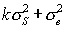Within Subj n(k - 1)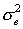Partner     Error k    (n-1)(k-1) ---    ---

If each of the members of a couple had exactly the same score, there would be no within-subject variance, and all of the variance in the experiment would be due to differences between subjects. (Remember, we are using the analysis of variance terms "between subjects" or within subjects" to refer to what we would really think of as "between couples" and "within couples.") We can therefore obtain a measure of the degree of relationship by asking what proportion of the variance is between subjects variance. Thus we will define our estimate of the correlation as the intraclass correlation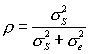Just to amuse myself, at the risk of losing the reader, I will actually derive the estimate of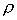.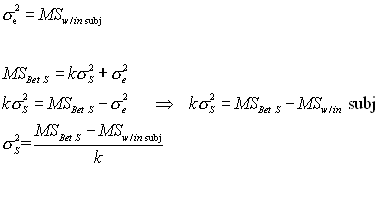Now I have the estimates for the terms in my formula for the estimate of. I simply substitute them into the formula.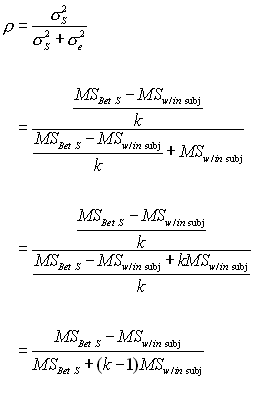And, for the case with k = 2 observations per couple, the k - 1 term drops out.

An important thing to notice here is that MSw/in subj will not be affected by which score you put in column 1, and which goes in column 2. The variance of 45 and 49 is exactly the same as the variance of 49 and 45. Thus the order of assignment is irrelevant to the statistical result, which is exactly what we want.

The derivation above, which is essentially the same as that of  Shrout and Fleiss (1979), leads to the following formula for the intraclass correlation coefficient.Notice that I keep hopping back and forth between "couple," which is the term we would use from our example, and "subject," which is the way the analysis of variance would refer to this effect.

## Example

I have created a set of data for 50 couples that resembles the example above. These data are available at PartnerCorr.dat or at PartnerCorr.sav.  I will set up the analysis in SPSS as a repeated measures analysis of variance, though I will completely ignore the effect due to Partners.  (The breakdown to Partners is only needed so that I can add the components back together to get a within-subjects term.

General Linear ModelI will set this up in a more traditional summary table. However, in a traditional table the term that we have labeled "Couple" is normally called "Subjects," and that is the notation that I will use here. Notice that the only reason for having a Partner and Error term is to allow be to add them together to obtain the Within Subjects term. Also note that what SPSS calls the Error term in the Between- Subjects part of the table is what we would normally call the Between Subjects term. With these changes we obtain the following table.

 Source df SS MS F Between Subj 49 14113.810 288.037 Within Subj 50 1567.500 31.35 Partner     Error 1    49 13.690     1553.810 13.690 31.710

From the formula given above we have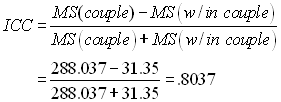Thus our estimate of the correlation of sociability scores between partners in gay couples is .80. (These are fictitious data, and I don't know what the true correlation would be.)

One plea of ignorance.

Notice that my estimate of the ICC is a ratio of the variance between subjects over the total variance. But from what you probably already know, you should expect that this would be a squared correlation. Look back to the formula for eta-squared, for example. But here we treat this as a regular correlation, not as a squared one. I have to come straight out and admit that I don't understand why that is so. I can't explain why the formula above is expressed as a formula for, rather than a formula for2 . But I am quite sure that Shrout and Fleiss are correct here. This issue arises frequently in the reliability literature.

### An easier way

If you are using SPSS to analyze your data, there is an easier way to calculate this coefficient. The advantage of this approach is that it also produces a confidence interval on our estimate.

The procedure that we want is the Reliability procedure, which is an old procedure in SPSS that has not been rewritten (at least in version 10) to use the more modern display of output. However it can be invoked from the menu structure.

First chose Analysis/Scale/Reliability Analysis from the menu. That will produce the following dialog box.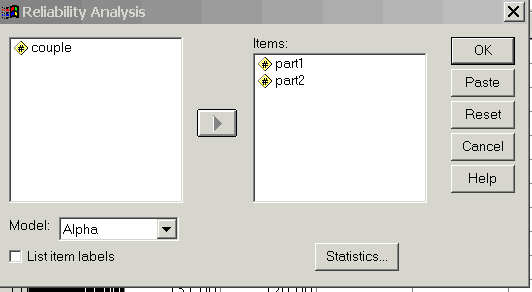Notice that I have included the two variables (Partner1 and Partner2). You next need to click on the Statistics box, which will give you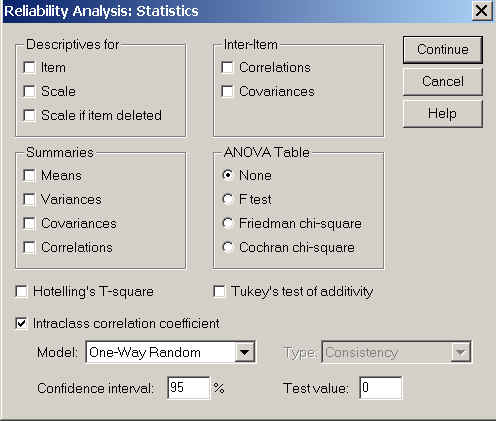Here I have selected the intraclass correlation coefficient, and then selected the One-Way Random model. (That is important--you don't want to take the default option.)

The results are shown below.

********************************************************* ******************

****** Method 1 (space saver) will be used for this analysis ******

R E L I A B I L I T Y A N A L Y S I S - S C A L E (A L P H A)

Intraclass Correlation Coefficient

One-way random effect model: People Effect Random

Single Measure Intraclass Correlation = .8037

95.00% C.I.: Lower = .6791 Upper = .8834

F = 9.1878 DF = ( 49, 50.0) Sig. = .0000 (Test Value = .0000 )

Average Measure Intraclass Correlation = .8912

95.00% C.I.: Lower = .8089 Upper = .9381

F = 9.1878 DF = ( 49, 50.0) Sig. = .0000 (Test Value = .0000 )

Reliability Coefficients

N of Cases = 50.0 N of Items = 2

Alpha = .8899

********************************************************* ************************

Here you can see that the intraclass correlation agrees perfectly with the measure that we calculated (.8037). The "Average Measure Intraclass Correlation" is not relevant to this particular problem. It represents our estimate of the reliability if we averaged the scores of the two partners, and used that as a variable. It can be obtained directly from the intraclass correlation coefficient by using the Spearman-Brown Prophecy formula (rSB = [(2*ricc)/(1+ricc)].

## Other forms of the intraclass correlation

As I said at the beginning, there are several different kinds of intraclass correlation coefficients. The one discussed here is used for twin studies or any other studies where there is no way of assigning members within a pair to a specific variable--or, as denoted in the subtitle of this page, where the scores within a pair are not ordered. I may eventually put together a page on other coefficients, but not today.

### Cited references

Griffin, D., & Gonzalez, R. (1995). Correlational analysis of dyad-level data in the exchangeable case. Psychological Bulletin, 118, 430-439

Shrout, P.E. & Fleiss, J.L. (1979) Intraclass Correlations: Uses in Assessing Rater Reliability. Psychological Bulletin, 2, 420-428.

### Additional references of interest

Field, A. P. (2005) Intraclass correlation. In Everitt, B. S. & Howell, D.C. Enclyopedia of Statistics in Behavioral Sciences . Chichester, England; Wiley. McGraw, K. O. & Wong, S. P. (1996. Forming inferences about some intraclass correlation coefficients. Psychological Methods, 1, 30 - 46.

A good program for carrying out the calculations of intraclass coefficients in R or S-Plus can be found in the irr package, which can be downloaded from the R site.Return to Dave Howell's Statistical Home Page

Send mail to: David.Howell@uvm.edu)